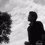# Solution for previous proposal

Hello Brilliants, here I wish to share my solution for the proposed problem.

Before we start with the problem we shall consider the integral of the form for all $n\geq 1$ $I(n) = \displaystyle \int_0^1 x^{n-1} \ln(1-x) dx =\frac{1}{n}\displaystyle \int_0^1 \frac{d}{dx}(x^{n}-1)\ln(1-x) dx$ and by integration by parts we yield $I(n)=\frac{1}{n}\left[\underbrace{(x^{n}-1)\ln(1-x)}_{0}\right]_0^1-\frac{1}{n}\int_0^1\frac{1-x^{n}}{1-x}dx$$=-\frac{1}{n}\int_0^1\sum_{j=1}^{n} x^{j-1} =-\frac{1}{n}\sum_{j=1}^{n}\int_0^1 x^{j-1}dx=-\frac{1}{n} \sum_{j=1}^n\frac{1}{j} =-\frac{H_n}{n}\cdots (1)$ Note that $D(k)=\displaystyle \frac{1}{n^2m+m^2n+kmn} = \frac{1}{mn}\int_0^1 x^{m+n+k-1} dx=\frac{x^{k-1}}{mn}\int_0^1{x^{m+n}}dx$ and therefore $\sum_{1\leq n\leq m\leq \infty} D(k)=\int_0^1 x^{k-1} \sum_{n=0}^{\infty}\sum_{m=0}^{\infty} \frac{x^n}{n}\cdot \frac{x^m}{m}dx =\int_0^1 x^{k-1}\ln^2(1-x) dx$ Now we consider the latter integral $I(k)=\displaystyle \int_0^1 x^{k}\ln^2(1-x) dx$ for $k\geq 0$ which further can be written as $\displaystyle \frac{1}{k+1}\int_0^1 \frac{d}{dx}(x^{k+1}-1)\ln^2(1-x) dx$ and hence on Integration by parts we see that $I(k)=\frac{1}{k+1}\left[\underbrace{(x^{k+1}-1)\ln^2(1-x)}_{0}\right]_0^1-\frac{2}{k+1}\int_0^1\frac{1-x^{k+1}}{1-x}\ln(1-x)dx$$=-\frac{2}{k+1}\int_0^1\sum_{n=1}^{k+1} x^{n-1}\ln(1-x)=-\frac{2}{k+1}\sum_{n=1}^{k+1}\int_0^1 x^{n-1}\ln(1-x)dx$ Plugging the result from $(1)$ to last integral we have $\frac{2}{k+1}\sum_{n=1}^{k+1}\frac{H_n}{n}=\frac{2}{k+1}\left(\frac{H_{k+1}^2+H_{k+1}^{(2)}}{2}\right)=\frac{H_{k+1}^2 +H_{k+1}^{(2)}}{k+1}$ Further note that nth partial sum of $H_{k+1}^{(2)} = \zeta(2) -\psi^1(k+2)$ giving us required result of $D(k)=\frac{H_{k+1}^2-\psi^1(k+2)}{k+1}+\frac{\pi^2}{6(k+1)}$

Now we notice that $\frac{1}{(q+b)\sqrt{q+b+1}+(q+b+1)\sqrt{q+b}}=\frac{(q+b)\sqrt{q+b+1}-(q+b+1)\sqrt{q+b}}{(q+b)\sqrt{q+b+1}(-1)}$$=\frac{1}{\sqrt{q+b}}-\frac{1}{\sqrt{q+b+1}}$ and hence $\sum_{n=0}^{\infty}\sum_{q=0}^n x^n\left(\underbrace {\frac{1}{\sqrt{q+b}}-\frac{1}{\sqrt{q+b+1}}}_{\text{Telescoping}}\right)=\sum_{n=0}^{\infty}x^n\left(\frac{1}{\sqrt{b}}-\frac{1}{\sqrt{n+b+1}}\right)$ and by hence by algebra of sum and for for $|x|<1$ $\sum_{n=0}^{\infty}\frac{x^n}{\sqrt{b}}-\sum_{n=0}^{\infty}\frac{x^n}{\sqrt{n+b+1}}=\frac{1}{\sqrt{b}(1-x)}-\Phi\left(x, \frac{1}{2},1\right)$ and we deduce that $\alpha = 2, \beta =1 ,\gamma =6$. Thus $\Phi\left(\beta-\alpha,\beta , (\alpha+2\beta \gamma)^{-1}\right)=\Phi\left(-1,1,\frac{1}{10}\right)=\sum_{n=0}^{\infty}\frac{(-1)^{n}}{\left(n+\frac{1}{10}\right)}=D$ $D= \sum_{n=0}^{\infty}\frac{1}{\left(2n+10^{-1}\right)(2n+10^{-1}+1)}=\frac{1}{4}\sum_{n=0}^{\infty}\frac{1}{(n+20^{-1})(n+20^{-1}+2^{-1})}$ $=2^{-1}\left(\psi\left(\frac{1}{2}+\frac{1}{10}\right)-\psi\left(\frac{1}{20}\right)\right)=2^{-1}\left(\psi\left(\frac{1}{10}\right)-2\psi\left(\frac{1}{20}\right)-2\ln 2\right)$and finally we have $\displaystyle D=\psi\left(\frac{1}{10}\right)-\psi\left(\frac{1}{20}\right)-\ln 2$. Here to evaluate this expression $D$ , we use the Gauss's-Diagamma theorem ie $\psi\left(\frac{r}{m}\right)=-\gamma -\ln(2m)-\frac{\pi}{2}\cot\left(\frac{r\pi}{m}\right)+2\sum_{q=1}^{\left\lfloor \frac{m-1}{2}\right\rfloor}\cos\left(\frac{2\pi qr}{m}\right)\ln\sin\left(\frac{\pi q}{n}\right)$ plugging $r=1, m=10 ,20$ we have $I= \psi\left(\frac{1}{10}\right)=-\gamma -\ln(20)-\frac{\pi}{2}\cot\left(\frac{\pi}{10}\right)+2\sum_{q=1}^{4}\cos\left(\frac{\pi q}{5}\right)\ln\sin\left(\frac{\pi q}{10}\right)$ and $K= \psi\left(\frac{1}{20}\right)=-\gamma -\ln(40)-\frac{\pi}{2}\cot\left(\frac{\pi}{20}\right)+2\sum_{q=1}^{9}\cos\left(\frac{\pi q}{10}\right)\ln\sin\left(\frac{\pi q}{20}\right)$ and also $D=I-k -\ln 2$ and on further simplification of the $D$ we obtain that $D = \frac{\pi}{4}\frac{1}{\sin\left(\frac{1}{20}\right)\cos\left(\frac{1}{20}\right)}+A\left(\log\cot\left(\frac{\pi}{20}\right)\right)+B\left(\log\cot\left(\frac{3\pi}{20}\right)\right)$ with $A=\sqrt{\frac{5+\sqrt 5}{2}}=\sqrt{\phi+2}$ and $B=\sqrt{\frac{5-\sqrt{5}}{2}}=2^{-1}\sqrt{10-2\sqrt{5}}$ and $\displaystyle \frac{\pi}{4}\frac{1}{\sin\left(\frac{\pi}{20}\right)\cos\left(\frac{\pi}{20}\right)}\\ =\frac{\pi}{2}\frac{1}{\sin(18^{\circ})}=\frac{2\pi}{\sqrt 5-1}$ since $\sin(18^{\circ} )=4^{-1}\left(\sqrt 5-1\right)$.

Further we have $\displaystyle\cot\left(\frac{\pi}{20}\right)=\cot(9^{\circ})$. To evaluate $\cot(9^{\circ}$ we shall be using the fact that $\cot (3x)=\frac{\cos (3x)}{\sin (3x)}= \frac{4 \cos^3 x-3\cos x}{3\sin x-4\sin^3 x}=\frac{\cos x(1-4\sin ^2 x)}{\sin x(3-4\sin^2 x)}=\cot x\left(1-\frac{2}{3-4\sin^2 x}\right)$ setting $x=3$ we have the expression for $\cot9^{\circ}$ but then we will show that $\sin 3^{\circ}=\frac{1}{4}\sqrt{8-\sqrt{10-2\sqrt 5}-\sqrt{15}-\sqrt{3}}$ {Note}: This identity is proposed by Narendra Bhandari and is proved by Sergio Esteban beautiful geometry work) and Ahmed Hegazi(trigonometry work). From half angle formula we have that $\sin\frac{\phi}{2}=\sqrt{\frac{1-\cos \phi}{2}}$ now set $\phi = 6^{\circ}$. Giving us $\sin3^{\circ} =\sqrt{\frac{1-\cos 6^{\circ}}{2}}= \sqrt{\frac{1-\cos(36^{\circ} -30^{\circ})}{2}}$ Now using compound angle formula we can get
$\displaystyle \cos(36^{\circ} -30^{\circ}) = \cos36^{\circ}\cos30^{\circ}+\sin36^{\circ}\sin30^{\circ} \\= \left(\frac{\sqrt 5 +1}{4} \right) \frac{\sqrt 3}{2} +\left(\frac{\sqrt{10-2\sqrt5}}{4}\right)\frac{1}{2} =\frac{\sqrt{10-2\sqrt 5}+\sqrt 3 +\sqrt {15}}{8}$putting in original equation $\sin3^{\circ} =\frac{1}{\sqrt 2} \left( \sqrt{1- \frac{\sqrt{10-2\sqrt 5}+\sqrt 3 +\sqrt {15}}{8} }\right) = \frac{1}{4}\left(\sqrt{8-\sqrt{10-2\sqrt 5}-\sqrt 3 -\sqrt {15} }\right)$ and hence $\cos 3^{\circ} = \sqrt{1-\sin^2 3^{\circ}} =\frac{1}{4}\sqrt{8+\sqrt{10-2\sqrt 5} +\sqrt{10}+\sqrt{3}}$ and hence we deduce easily that $\cot9^{\circ}= \cot3^{\circ}\left(1-\frac{8}{4+\sqrt{10-2\sqrt 5}+\sqrt{15}+\sqrt{3}}\right)$ also $\cot\left(\frac{3\pi}{20}\right)=\cot(27^{\circ}=\cot(30-3)$ and using compound angle formula for $cot(a-b)$ we have $\cot27^{\circ}= \frac{\cot 3^{\circ} \cot30^{\circ}-1}{\cot 3^{\circ}+\cot 30^{\circ}}=\frac{\sqrt 3 \cot3^{\circ} -1}{\cot3^{\circ}+\sqrt 3}$ and hence we have $D=\Phi(\beta-\alpha,\beta , (\alpha +2\beta +\gamma)^{-1}$ $=\frac{2\pi}{\sqrt5 -1}+\sqrt{\phi+2}\log\left(\theta -\frac{8\theta}{4+\sqrt{10-2\sqrt 5}+\sqrt{15}+\sqrt {3}}\right)+2^{-1}\sqrt{10-2\sqrt 5}\log\left(\frac{\sqrt 3 \theta -1}{\theta +\sqrt 3}\right)$ and thus $\displaystyle \theta=\frac{\sqrt{8+\sqrt{10-2\sqrt{5}}+\sqrt{15}+\sqrt{3}}}{\sqrt{8-\sqrt{10-2\sqrt{5}}-\sqrt{15}-\sqrt{3}}}$ we are done

Other different approaches are highly appreciated. I wish to be corrected if any such typos or errors are spotted in the above solution. Thank you!Note by Naren Bhandari
1 year, 2 months ago

This discussion board is a place to discuss our Daily Challenges and the math and science related to those challenges. Explanations are more than just a solution — they should explain the steps and thinking strategies that you used to obtain the solution. Comments should further the discussion of math and science.

When posting on Brilliant:

• Use the emojis to react to an explanation, whether you're congratulating a job well done , or just really confused .
• Ask specific questions about the challenge or the steps in somebody's explanation. Well-posed questions can add a lot to the discussion, but posting "I don't understand!" doesn't help anyone.
• Try to contribute something new to the discussion, whether it is an extension, generalization or other idea related to the challenge.
• Stay on topic — we're all here to learn more about math and science, not to hear about your favorite get-rich-quick scheme or current world events.

MarkdownAppears as
*italics* or _italics_ italics
**bold** or __bold__ bold
- bulleted- list
• bulleted
• list
1. numbered2. list
1. numbered
2. list
Note: you must add a full line of space before and after lists for them to show up correctly
paragraph 1paragraph 2

paragraph 1

paragraph 2

[example link](https://brilliant.org)example link
> This is a quote
This is a quote
    # I indented these lines
# 4 spaces, and now they show
# up as a code block.

print "hello world"
# I indented these lines
# 4 spaces, and now they show
# up as a code block.

print "hello world"
MathAppears as
Remember to wrap math in $$ ... $$ or $ ... $ to ensure proper formatting.
2 \times 3 $2 \times 3$
2^{34} $2^{34}$
a_{i-1} $a_{i-1}$
\frac{2}{3} $\frac{2}{3}$
\sqrt{2} $\sqrt{2}$
\sum_{i=1}^3 $\sum_{i=1}^3$
\sin \theta $\sin \theta$
\boxed{123} $\boxed{123}$

## Comments

Sort by:

Top Newest

Man I didn't understood the step where you pull out the x to the k-1 variable out from the integral.

- 1 year ago

Log in to reply

I don't know calculus but I like your solutions (both of them!)

- 1 year, 1 month ago

Log in to reply

I'm glad to know, you like it.Thank you :)

- 1 year, 1 month ago

Log in to reply

×

Problem Loading...

Note Loading...

Set Loading...